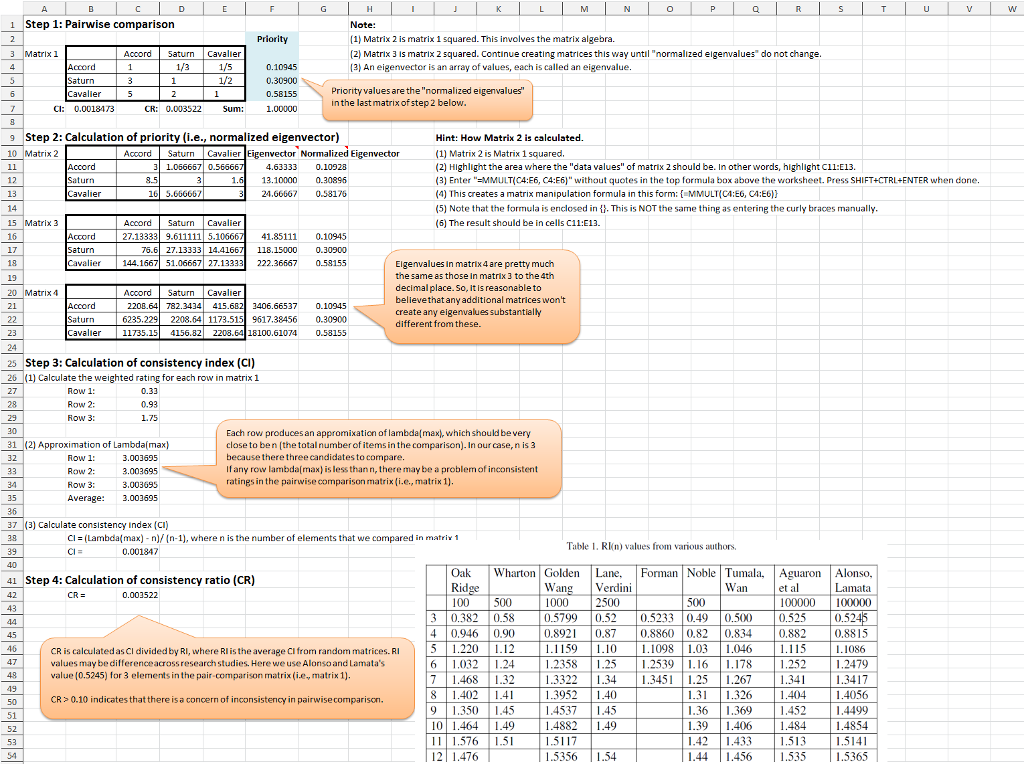# Solved: Use the pairwise comparison matrix for the price criterion as shown in Table 14.8 to verify

Use the pairwise comparison matrix for the price criterion as shown in Table 14.8 to verify that the priorities after synthesization for price are 0.110, 0.309, and 0.581. Compute the consistency ratio and comment on its acceptability. Round your answers to three decimal places. Lambda_max = Consistency Index = Consistency Ratio = Since the consistency ratio is than, the degree of consistency in the pairwise comparison matrix for price acceptable.λmax = 3.003695

Don't use plagiarized sources. Get Your Custom Essay on
Solved: Use the pairwise comparison matrix for the price criterion as shown in Table 14.8 to verify
GET AN ESSAY WRITTEN FOR YOU FROM AS LOW AS \$13/PAGE

Consistency Index = 0.001847

Consistency Ratio = 0.003522

Since the consistency ratio is less than 0.10, the degree of consistency in the pairwise comparision matrix for price is acceptable.